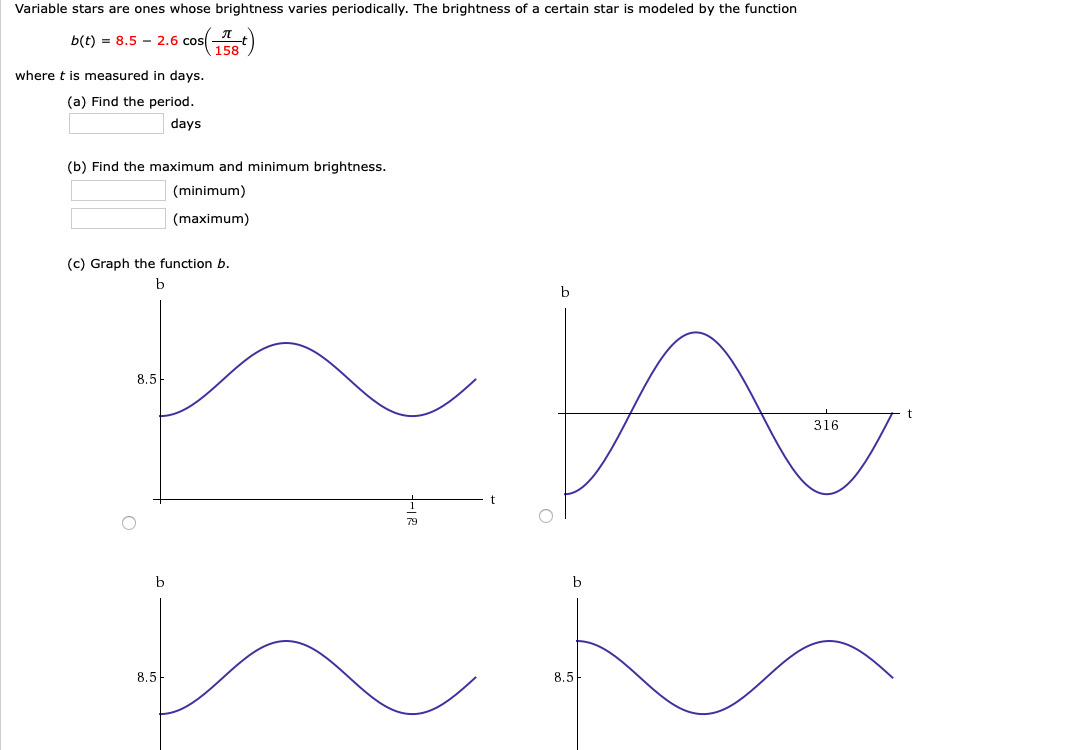# Variable stars are ones whose brightness varies periodically. The brightness of a certain star is modeled by the functionb(t) = 8.5 – 2.6 cos( t)158where t is measured in days.(a) Find the period.days(b) Find the maximum and minimum brightness.(minimum)(maximum)(c) Graph the function b.8,5316798.5-8.5

Question
19 viewshelp_outlineImage TranscriptioncloseVariable stars are ones whose brightness varies periodically. The brightness of a certain star is modeled by the function b(t) = 8.5 – 2.6 cos( t) 158 where t is measured in days. (a) Find the period. days (b) Find the maximum and minimum brightness. (minimum) (maximum) (c) Graph the function b. 8,5 316 79 8.5- 8.5 fullscreen
check_circle

Step 1

To find the period we divide 2pi by coefficient of t.

Step 2

cos theta lies between -1 to 1.

We use that to find max and min.

### Want to see the full answer?

See Solution

#### Want to see this answer and more?

Solutions are written by subject experts who are available 24/7. Questions are typically answered within 1 hour.*

See Solution
*Response times may vary by subject and question.
Tagged in

### Calculus